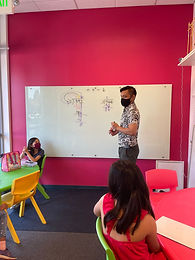## Mr. Rodney

### Target 1​

###### Lesson Type:

New

Data Probability

:

Ratio

Understand ratio concepts and use ratio reasoning to solve problems.

###### 1:

Understand that a ratio represents a comparison of two quantities.

5th

###### Vocabulary:

Ratio, Weight

Activities:

• Students explored and experimented with various objects and quantities to balance out the scales, and their ratios in weight.
• Used the information given by 2 ratios to solve for a third. For example, if 1 bolt = 3 bananas, and 2 bananas = 8 cubes, how many cubes equals 1 bolt?
• Practiced reducing and simplifying ratios (unknowingly)### Home Exploration

###### Guiding Questions:## Absent Students:

Amisha (2nd half)

### Target 2

:

###### 1:

Identify in a given problem what the unknown information is.

###### 2:

Read, write, and evaluate expressions in which letters stand for numbers.

5th

###### Vocabulary:

Variable, Value, Long Division, Decimal

Activities:

• Reviewed steps and procedure required to solve a long division problem, dividing multi-digit whole numbers as well as decimals.
• Studied a grid filled with symbols or "variables," in which each figure represents and unknown value, but the sum of each row and column is given.
• Used the information given to calculate the value of each symbol.### Home Exploration

###### Guiding Questions:### Target 3

:

###### Vocabulary:

Activities:### Home Exploration